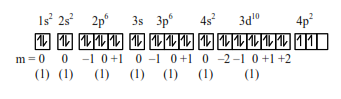# Solve this followingQuestion:

$\operatorname{Ge}(Z=32)$ in its ground state electronic configuration has x completely filled orbitals with $\mathrm{m}_{l}=0 .$ The value of $\mathrm{x}$ is

Solution:Completely filled orbital with $\mathrm{m}_{t}=0$ are

$=1+1+1+1+1+1+1$

$=7$